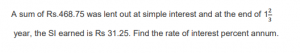# Simple Interest

• Last Updated : 27 Sep, 2023

 Question 1

A sum amounts to Rs 1065 at simple interest rate of 7.5% per annum after 3 years. Find the sum.878.4869.38869.28783.2

Question 1-Explanation:

Let the required sum be x. Then,

Simple interest = (x × 3 × 7.5 /100) = Rs 22.5x/100.

Amount = (x + 22.5x/100) = Rs 122.5x/100.

Therefore, 122.5x/100 = 1065 ⇒ 122.5x = 1065 × 100 ⇒ x = 106500/122.5 ⇒ x = 869.38

 Question 2

James deposits a sum of Rs 800 that will amount to Rs 920 in 3 years. However, the bank decides to increase the interest rate by 4%. What amount will he receive now?Rs 1015Rs 1016Rs 1025Rs 1102

Question 2-Explanation:

James gets simple interest (SI) = Rs 920-800 = Rs 120.

Here, Principal (P) = Rs 800 and Time (T) = 3 years.

We know, Rate (R) = (100×SI)/(P×T) = (100×120)/(800×3) = 5%.

Given, New Rate = 9%. So, New Interest = P×R×T = 800×0.09×3 = Rs 216.

Therefore, James will get Rs (800+216) = Rs 1016.

 Question 3
What will be the simple interest on Rs 70,000 at 50/3 % per annum after 9 months?Rs 8750Rs 8745Rs 8678Rs 8555

Question 3-Explanation:
Given, Principal (P) = Rs 70000, Rate (R) = 50/3 % per annum and Time (T) = 9/12 = 3/4 years. Simple Interest (SI) = P×R×T = 70000×(50/300)×(3/4) = Rs 8750.
 Question 4

Find out the simple interest if Harry invests an amount of Rs 15800 for 9 months at the rate of 25/4 % per annum.Rs 740.54Rs 745.63Rs 742.63Rs 740.63

Question 4-Explanation:

Given, Principal = 15800. Rate = 25/4 % per annum. Time = 9/12 = 3/4 years. Hence, SI = 15800×(25/400)×(9/12) = Rs 740.63.

 Question 5

After lending out an amount of Rs.9600 at a rate of 9/2 % per annum for a 1 year and 9 months, what was the S.I earned ?677756765625

Question 5-Explanation:

Given that, principal = P = Rs.9600, R = 9/2 % and T = 1 year and 9 months = 1 + 9/12 year = 7/4 years.
Now, we have to find the S.I for 7/4 years.
S.I = PRT/100 = Rs. 9600 x 9/2 x 7/4 x 1/100 = 12 x 9 x 7 = 756
Hence, the required S.I amount is Rs.756

 Question 6

Find the amount of S.I received by Ram after getting Rs.3360 at 25/4 % per annum for 9 months.156.5153.2168.5157.5

Question 6-Explanation:

S.I = PRT/100
= Rs. 3360 x 25/4 x 3/4 x 1/100 = 21 x 25 x 3
= Rs.157.5

 Question 7
Ram has an amount of Rs. 9000. How much time will it take to earn 1620 as S.I at a rate of 4.5% per annum?2154

Question 7-Explanation:
SI = PRT/100 T = S.I x 100/PR = 1620 x 100/9000 x 4.5 = 4
 Question 8
At what rate of SI, a sum of Rs.7000 amounts to Rs.7700 in 2 years?5.5%6.5%6%5%

Question 8-Explanation:
SI= 7700-7000 = 700 700 = 7000*R*2/100 R = 70000/14000 = 5%
 Question 9
Find the simple interest on Rs.300 for 6 months at 10% per annum.20151713

Question 9-Explanation:
SI = 300*10*(6/12)/100
= 15
 Question 10544.256

Question 10-Explanation:
R=SI. 100/P.T = (31.25 X 100)/(468.75 X 5/3) = 4
There are 15 questions to complete.